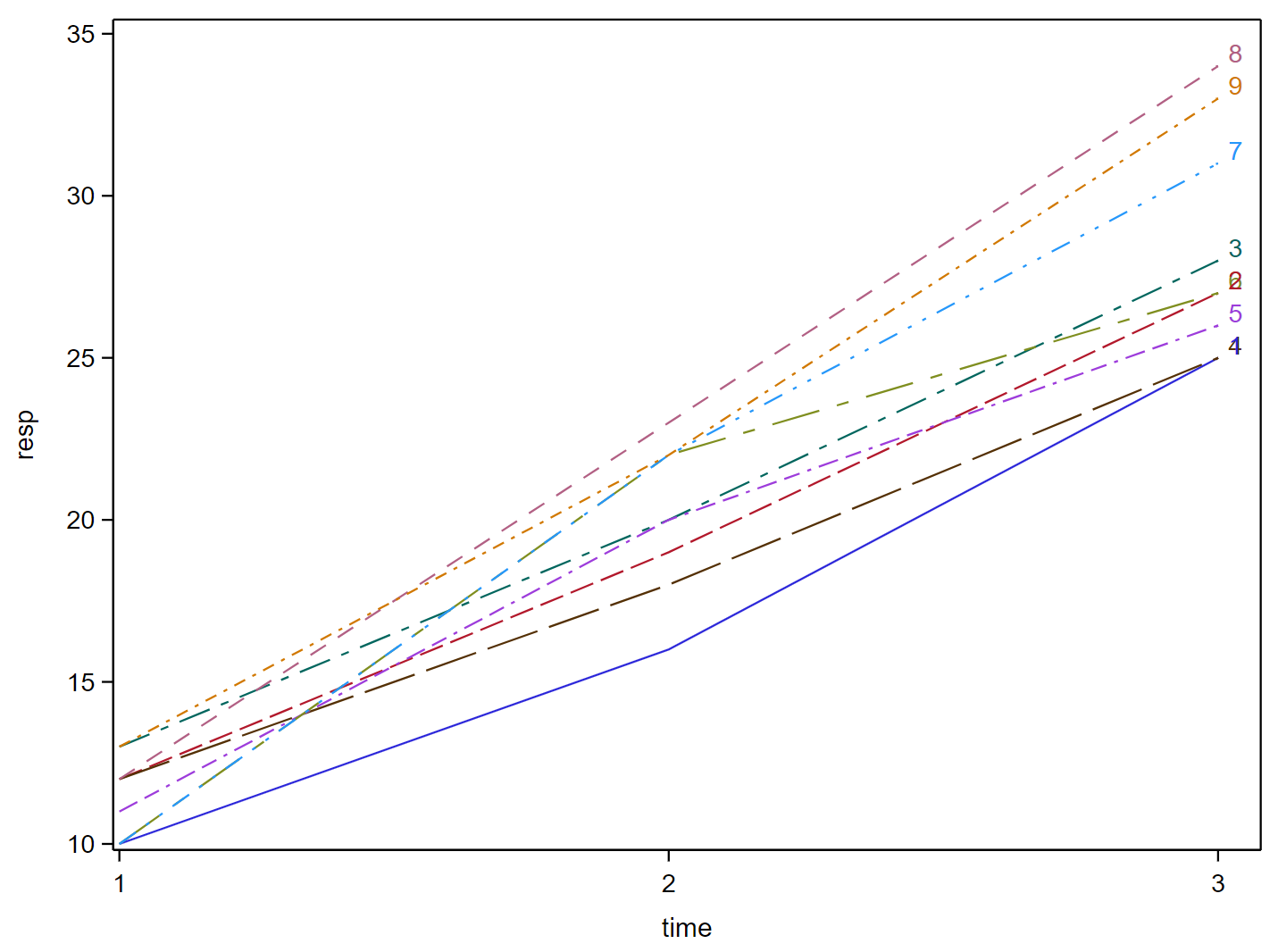# 11.4: Worked Example

$$\newcommand{\vecs}{\overset { \rightharpoonup} {\mathbf{#1}} }$$ $$\newcommand{\vecd}{\overset{-\!-\!\rightharpoonup}{\vphantom{a}\smash {#1}}}$$$$\newcommand{\id}{\mathrm{id}}$$ $$\newcommand{\Span}{\mathrm{span}}$$ $$\newcommand{\kernel}{\mathrm{null}\,}$$ $$\newcommand{\range}{\mathrm{range}\,}$$ $$\newcommand{\RealPart}{\mathrm{Re}}$$ $$\newcommand{\ImaginaryPart}{\mathrm{Im}}$$ $$\newcommand{\Argument}{\mathrm{Arg}}$$ $$\newcommand{\norm}{\| #1 \|}$$ $$\newcommand{\inner}{\langle #1, #2 \rangle}$$ $$\newcommand{\Span}{\mathrm{span}}$$ $$\newcommand{\id}{\mathrm{id}}$$ $$\newcommand{\Span}{\mathrm{span}}$$ $$\newcommand{\kernel}{\mathrm{null}\,}$$ $$\newcommand{\range}{\mathrm{range}\,}$$ $$\newcommand{\RealPart}{\mathrm{Re}}$$ $$\newcommand{\ImaginaryPart}{\mathrm{Im}}$$ $$\newcommand{\Argument}{\mathrm{Arg}}$$ $$\newcommand{\norm}{\| #1 \|}$$ $$\newcommand{\inner}{\langle #1, #2 \rangle}$$ $$\newcommand{\Span}{\mathrm{span}}$$$$\newcommand{\AA}{\unicode[.8,0]{x212B}}$$

For the example dataset in Repeated Measures Example Data, which we introduced in the 11.2: Correlated Residuals section, we can plot the data:Figure $$\PageIndex{1}$$: The values of the response plotted at each of the three time points for each of the 9 subjects.

We can obtain the results for the split-plot in time approach using the following:

/* Split-Plot in Time */
proc mixed data=rmanova method=type3;
class trt time subject;
model resp=trt time trt*time / ddfm=kr;
random subject(trt); title 'Split-Plot in Time';
run;


Next, we run the analysis as a repeated-measures ANOVA, which allows us to evaluate which covariance structure fits best.

Next, we run the analysis as a repeated-measures ANOVA, which allows us to evaluate which covariance structure fits best.

/* Repeated Measures Approach */
/* Fitting Covariance structures: */
/* Note: the code begining with "ods output ..." for each
run of the Mixed procedure generates an output that
is tabulated at the end to enable comparison of
the candidate covariance structure */
proc mixed data=rmanova;
class trt time subject;
model resp=trt time trt*time / ddfm=kr;
repeated time/subject=subject(trt) type=cs rcorr;

ods output FitStatistics=FitCS (rename=(value=CS))
FitStatistics=FitCSp;
title 'Compound Symmetry'; run;
title ' '; run;

proc mixed data=rmanova;
class trt time subject;
model resp=trt time trt*time / ddfm=kr;
repeated time/subject=subject(trt) type=ar(1) rcorr;

ods output FitStatistics=FitAR1 (rename=(value=AR1))
FitStatistics=FitAR1p;
title 'Autoregressive Lag 1'; run;
title ' '; run;

proc mixed data=rmanova;
class trt time subject;
model resp=trt time trt*time / ddfm=kr;
repeated time/subject=subject(trt) type=un rcorr;

ods output FitStatistics=FitUN (rename=(value=UN))
FitStatistics=FitUNp;
title 'Unstructured'; run;
title ' '; run;

data fits;
merge FitCS FitAR1 FitUN;
by descr;
run;
ods listing; proc print data=fits; run;


We get the following Summary Table:

Obs Descr CS AR1 UN
1 -2 Res Log Likelihood 70.9 71.9 63.0
2 AIC (smaller is better) 74.9 75.9 75.0
3 AICC (smaller is better) 75.7 76.7 82.6
4 BIC (smaller is better) 75.3 76.3 76.2

Using the AICC as our criteria, we would choose the compound symmetry (CS) covariance structure.

The output from this would be:

Type 3 Test of Fixed Effect
Effect Num DF Den DF F Value Pr > F
trt 2 6 7.14 F">0.0259
time 2 12 605.62 F">< .0001
trt*time 4 12 9.66 F">0.0010

Note that for this case, the $$p$$-values obtained here are identical to the split-plot in time approach.

##### Using R
Steps in R

1. Obtain the results for the split-plot in time approach by using the following commands:

library(lmerTest)
library(lme4)
model<-lmer(resp ~ trt + factor(time) + trt:factor(time) + (1 | factor(subject) : (trt)) , repeated_measures_example_data)
anova(model)
#Type III Analysis of Variance Table with Satterthwaite s method
#               Sum Sq  Mean Sq  NumDF  DenDF  F value   Pr(>F)
#trt                15        8      2      6     7.14  0.02590 *
#(time)           1301      650      2     12   605.62  8.9e-13 ***
#trt:factor(time)   41       10      4     12     9.66  0.00099 ***
#---
#Signif. codes: 0 ‘***’ 0.001 ‘**’ 0.01 ‘*’ 0.05 ‘.’ 0.1 ‘ ’ 1


2. Run the analysis as a repeated-measures ANOVA by using different covariance structures. We can use the following commands:

library(nlme)
model_cs<-gls(resp ~ trt + factor(time) + trt*factor(time),repeated_measures_example_data,correlation=corCompSymm(form=~1|subject),method="ML")
model_AR<-gls(resp ~ trt + factor(time) + trt*factor(time),repeated_measures_example_data,correlation=corAR1(form=~1|subject),method="ML")
model_UN<-gls(resp ~ trt + factor(time) + trt*factor(time),repeated_measures_example_data,correlation=corSymm(form=~1|subject),method="ML")
Model_Selection <- data.frame(
c ("","-2LogLik","AIC", "BIC"),
c("CS", round(-2*summary(model_cs)$logLik,2),round(summary(model_cs)$AIC,2),round(summary(model_cs)$BIC,2)), c("AR1", round(-2*summary(model_AR)$logLik,2),round(summary(model_AR)$AIC,2),round(summary(model_AR)$BIC,2)),
c("UN", round(-2*summary(model_UN)$logLik,2),round(summary(model_UN)$AIC,2),round(summary(model_UN)\$BIC,2)),
stringsAsFactors = FALSE)
names(Model_Selection) <- c(" ", " ","","")
print(Model_Selection)
#1               CS     AR1      UN
#2 -2LogLik   80.54   82.03   69.95
#3      AIC  102.54  104.03   95.95
#4      BIC  116.79  118.29  112.79
detach(repeated_measures_example_data)


This page titled 11.4: Worked Example is shared under a CC BY-NC 4.0 license and was authored, remixed, and/or curated by Penn State's Department of Statistics via source content that was edited to the style and standards of the LibreTexts platform; a detailed edit history is available upon request.